### *(++p)++ and *++++p [问题点数：5分，结帖人waterluo2003]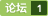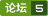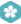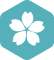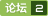&*p
char str = "hello";rn char *cp=str;rnrn cout<<
*p和**p的区别
int *p ：一级指针，表示p所指向的地址里面存放的是一个int类型的值 int **p ：二级指针，表示p所指向的地址里面存放的是一个指向int类型的指针（即p指向的地址里面存放的是一个指向int的一级指针） 例如： int i=10; //定义了一个整型变量 int *p=&i; //定义了一个指针指向这个变量 int **p1=&p; //定义了一个二级
*p和p[]的区别

free (p); p = NULL;
char *p = "Hello";rn free (p);rn p = NULL; rn printf("%s\n", (p) ? p : "NULL");rnrn为什么出现rnSegmentation fault (core dumped)rnrn将free（p）注释掉是正确的

(*p)[] 和　*p[]它们是一样的吗？如果不一样，那么它们的区别在哪里呢？
*p和p歪打正着了
char *p=new char;中 *p和p *(p+1)和p 所指针地址和地址里面的值都是一样的。 char *p=new char（10）;是将P这个地址里面的值值初始化为10； char *p=new char;  是声明一个是个元素的数组
p[]与*p的不同之处

char *p和char p[]

p[] 与 *p 的不同之处

p()和(*p)()的区别？

*p = 5; cout<<
#include "Stdio.h"rn#include rnint main(void)rnrn int *p=0;rn *p = 5;rn cout<<>a; rn return 0;rnrn在VC++ 6.0 中出现内存错误，这是为什么？
C语言*p、p以及&p的区别

1 #include&lt;iostream&gt; 2 #include&lt;iomanip&gt; 3 #include&lt;cmath&gt; 4 using namespace std; 5 int main() 6 { 7 int *p,i=1; 8 p=&amp;i; 9 cout&lt;&lt;"*p: "&lt;&lt;*...
* p
《C语言程序设计 现代方法》n第12章 指针和数组nn12.2 指针用于数组处理n*＋＋p或*(＋＋p)nn先自增p，自增后表达式的值是*pn—————————n书上这句话是什么意思？n实例：nint j=1,*p
*p 与**p 参数
1.原来指针和指针的指针这个老概念也不是很好理解的void GetMemory(char *p) { p = (char *)malloc(100); } void main(void) { char *str = NULL; GetMemory(str); strcpy(str, "hello world"); printf(str); } 以
*p与**p的区别
int *p ：一级指针，表示p所指向的地址里面存放的是一个int类型的值 int **p ：二级指针，表示p所指向的地址里面存放的是一个指向int类型的指针（即p指向的地址里面存放的是一个指向int的一级指针） 例如： int i=10; //定义了一个整型变量 int *p=&amp;i; //定义了一个指针指向这个变量 int **p1=&amp;p; //定义了一个二级指针指向p指针 那...
P + 1 == *(P + 1) ?

p,&amp;p,*p的区别与联系

1，*p++由于++和*同等优先级，结合方向为自右向左，因此它等价与*（p++）。先引用p的值，实现*p的运算，然后再使p自增1。2，*（p++）与*（++P）前者是先取*p的值，然后使p加1。后者是先使p加１，再取*p 。若p的初值为&amp;amp;a，输出*（P++），得到a的值。输出*（++p），得到a的值3，++（*P）表示p所指的元素加1，如果p = a , 则++（*p）相...
int *p（);int （*p)（）；
**指向函数的指针 int (*p) ();可以理解为调用函数，其中（*p）这个整体可以看作是函数名称，p代表函数的入口地址，c语言中（）就代表了函数，所以把 p用括号括在一起变作 (p)就代表了一个函数,例： #include &lt;stdio.h&gt; int main() { int num; int choice; int res; int *p = N...
p范数（p norm）

p[]与*p的区别

if(p)还是if(p != NULL)

*p 与p[] 指向的内存

*p++,(*p)++,*++p,++*p有什么不同？？
*p++#include #include #includevoid main() { int str[]={1,2,3,4,5}; int *p=str; //*p++; printf("%x\n",p); printf("%d\n",*p++); printf("%x\n",p); pr
*p,&p,p在c中的区别
http://blog.csdn.net/firefly_2002/article/details/8031424

1．掌握PPP 的基本配置步骤和方法。 2．掌握PAP、CHAP 的基本配置步骤和方法。 3．掌握对PAP、CHAP进行诊断的基本方法 1．配置路由器之间的PPP 连接。 2．配置、验证PAP过程。 3．配置、验证CHAP过程。
P一下。P一下。P一下。P一下。
P一下。P一下。P一下。P一下。P一下。P一下。P一下。P一下。P一下。P一下。P一下。
div p和div>p的区别
## 代码段落 &amp;lt;style&amp;gt; div span{color:red;} &amp;lt;/style&amp;gt; &amp;lt;div&amp;gt; &amp;lt;p&amp;gt; &amp;lt;span&amp;gt; Hello World! &amp;lt;/span&amp;gt; &amp;lt;/p&amp;gt; &amp;lt;span&amp;gt; Study Css &amp;lt;/span&amp;gt;

(*p)[n]和*P[n]解析。
#include void main() { float score[] = {{60,70,80,90},{56,89,67,88},{34,78,90,66}}; float *search(float(*pointer),int n); float *p; int i,m; printf("enter the number of student:"); scanf("%d",&m
pkzip -p与-P到底有什么区别？

free(p)与free(&p)的区别？

*的级别比++高，但是由于++是先用p的值然后再执行自加运算若是*++p，则是先进行p的自加后再执行*运算若是++*p，则先是进行*的运算
while（p）与while（*p）的区别
p=首个数据节点指针 while(p) { //操作 p=p-&amp;gt;next; } while(p) 等价于while(p !=0) 检查的是p值是不是0，或者是不是空指针，如果是，则跳出循环，不再继续循环体 而while(*p) 相当于while( *p != 0) 检查的是p所指向的地址中的数据是否为0，但前提是p不能为NULL...
int*p与int **p的用法

INT *P; SIZEOF(P)是多少？
WIN32是32位的地址吗？
**p和二维数组p有没有区别?
**p和二维数组p有没有区别?

P 2 P聊天工具项目

void（＊p）（）和void＊p（）区别

html中p标签字体居中的方法。首先p标签给出宽和高，呈块状显示，水平居中属性text-align: center;垂直居中line-height: px;这里的line-height值和你给p标签的高度值是同一个值。 系

#include &amp;lt;stdio.h&amp;gt; char *test() { char *p = &quot;hello world&quot;; return p; } int main() { char *p = test(); printf(&quot;%s\n&quot;, p); return 0; } 输出：hello world   #include &amp;lt;stdio.h&amp;gt; char...
20190118 *P和&P三者的区别
//(原)https://www.cnblogs.com/mgstone/p/5827419.html 假设我们定义一个指针p。 那么会经常使用到三个符号： 1，p； 2，p; 3，&amp;amp;p; 初学者经常会感到很迷茫，到底这三个符号表示什么？ 我们知道，p是一个指针变量的名字，表示此指针变量指向的内存地址，如果使用%p来输出的话，它将是一个16进制数。而p表示此指针指向的内存地址中存放的内容，...
(*p)与*p的区别
(*p)与*p的区别int p p是一个数组，此数组有3个元素，每个元素都是int类型，也就是指向整型数据的指针类型。int a=10,b=20,c=30; int*p={&a,&b,&c};而int(*p)中的p是一个指向数组的指针，此数组有3个int类型的元素。例如：int a={1,2,3}; 那么p就是指向这个数组a的指针。 int(*p)=&a

对于一个函数：void func(void); 我们通常可以定义一个这样的函数指针指向它：void (*p)(void) = func; 通过p调用func时，通常有两种写法： p();或者(*p)(); 围绕这两种写法，当初C89制定的时候曾经有过争论。(*p)();是一种旧式的规定，旧式规定圆括号左边必须具有“函数”类型，如果是指向函数的指针，那么必须加上*声明符。但C...

int *p[] 与int (*p)[] 问题

char *p[]和 char p[][]的问题
int main()rnrn char *p;rn char q;rn //p = 'a';这个赋值语句运行时报错rn p = "ok";rn q = 'b';rn return 0;rn
*p与(*p)的迷惑；

char* p 与char p[] 的区别？
char* p = "Hello Linux!";rn与rnchar p[] = "Hello Linux!";rn的区别是？rnrn是不是char* p = "Hello Linux!";相当于const char p[] = "Hello Linux!"; ？？？

char c='a',*p; 变量c的类型是char，它的初始值为'a'。变量p是个char类型的指针。 p=&c; 符号“&”是取地址操作符。表达式&c的值就是变量c在内存中的地址。c的地址被赋值给p。我们可以看成是p指向c。 printf("%c,%c",*p,*p+1); 格式‘“%c”用于把表达式按照字符的形式进行打印。符号“*”表示间接访问操作符。表达式*p的值就是p所指向的对
char p[]与char *p的区别

[code=C/C++] #include rnusing namespace std;rnint main()rnrn int *p=new int;rn *p=3; //我为什么改成p=3 就出错了？rn cout<<
&*p 和 p有什么分别？

char *p 与 char p[] 的区别？？
[code=C/C++]rn#include rnrn#include rnrnvoid main()rnrn char *p = "abcd"; //??程序在运行到 *(p+1) = 'a'; 时候出现内存读写错误。rn char p[] = "abcd"; //换成这种写法，程序可以正常运行。rnrn printf("*(p+1) = %c\n", *(p+1));rn *(p+1) = 'a';rn printf("*(p+1) = %c\n", *(p+1));rnrn return;rnrn//等待高手解答疑问？？？？谢谢rnrn[/code]
(NULL == p)&&(p == NULL)的区别???
(NULL == p)&&(p == NULL)的区别什么？？？ 只是为了降低首次写错代码的可能性吗？？？

char *p to unsigned char *p
char *p to unsigned char *p

[code=C/C++]rn#include"stdio.h"rnint sum(int x,int y)rnrn return x+y;rnrnrnvoid main(void)rnrn int S1=10,rn S2,rn *p_int;rnrn int (*p)();rnrn p_int=&S1;rn// printf("S1=%d,*S1=%d\n",S1,*S1);rn printf("p_int=%d,*p_int=%d\n",p_int,*p_int); //p_int与*p_int不同rnrn p=sum;rn printf("sum=%d,*sum=%d,p=%d,*p=%d\n",sum,*sum,p,*p); //这里为什么p与*p的值一样rn S1=(*p)(10,20);rn S2=p(30,40);rn printf("(*p)(10,20)=%d\np(30,40)=%d\n",S1,S2);rnrn[/code]rnrn这段程序打印出来的结果，p_int与*p_int的值不一样可以理解，为什么p与*p，sum与*sum的值一样，加*了不是要取值吗？rn
if(null!=p)和if(p!=null)区别？？
//声明变量rnParameterInfo prnif(null!=p)rn...rnif(p!=null)rn...rn这两者有什么区别？？？
delete [] p 与 delete p的区别？？

struct node *p 和node *p

K M P 算 法 . p p t
kMp算法kMp算法kMp算法kMp算法kMp算法kMp算法kMp算法kMp算法kMp算法kMp算法kMp算法kMp算法kMp算法kMp算法kMp算法kMp算法kMp算法kMp算法kMp算法kMp算法kMp算法kMp算法
p=for (p=s;*p!='\0';p++);有这样赋值的吗？
char *s="hello"rnchar *p;rnp=for (p=s;*p!='\0';p++);rn谁见过这样的赋值语句，编译也通不过呀！！
C语言中 *p++、*++p、++*p 、(*p)++的区别
A = 第一步 第二步   *p++ *p p++ 先取值，后指针++ A = *p； *++p ++p *(++p) 先指针++，后取值 A = *(++p)； ++*p *p (*p)+1 先取值，后值++ A = (*p)+1； (*...